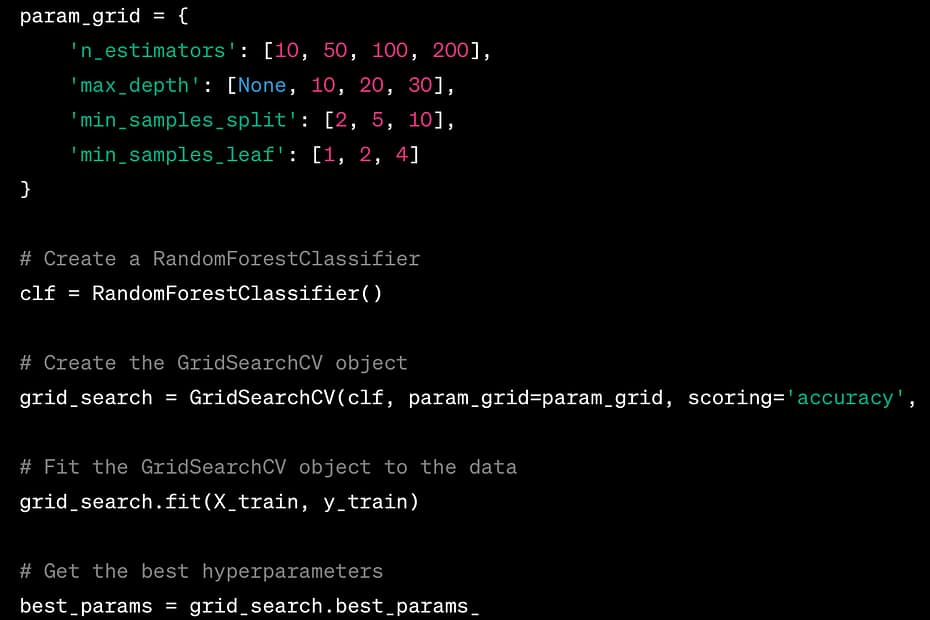# Tuning Scikit-Learn Hyperparameters: A Comprehensive GuideWhen it comes to building machine learning models with Scikit-Learn, choosing the right hyperparameters is often the key to achieving optimal performance. Hyperparameters are settings that are not learned from the data but must be specified before training begins. In this blog post, we will explore the importance of hyperparameter tuning and demonstrate three different techniques for tuning hyperparameters: manual tuning, RandomizedSearchCV, and GridSearchCV. We’ll also provide Jupyter notebook code snippets to help you implement these techniques.

## Why Hyperparameter Tuning Matters

Hyperparameters control various aspects of the machine learning algorithm’s behavior. Choosing the right values for these hyperparameters can significantly impact the model’s performance. Using the default hyperparameters may not lead to the best results for your specific problem, and that’s where hyperparameter tuning comes in.

## Manual Hyperparameter Tuning

Manual hyperparameter tuning involves adjusting hyperparameters based on your domain knowledge and intuition. While this approach can be effective, it is also time-consuming and requires a good understanding of the algorithm and the problem at hand. Here’s how you can do it:

``````from sklearn.ensemble import RandomForestClassifier

# Create a RandomForestClassifier with custom hyperparameters
clf = RandomForestClassifier(n_estimators=100, max_depth=10, min_samples_split=2, min_samples_leaf=1)

# Train the model
clf.fit(X_train, y_train)

# Evaluate the model
accuracy = clf.score(X_test, y_test)
``````

In this example, we manually set the `n_estimators`, `max_depth`, `min_samples_split`, and `min_samples_leaf` hyperparameters for a RandomForestClassifier. You would adjust these values and potentially other hyperparameters based on your understanding of the algorithm and problem.

## RandomizedSearchCV

RandomizedSearchCV is a more systematic approach to hyperparameter tuning. It randomly samples hyperparameters from predefined ranges, which can be more efficient than manual tuning. Here’s how to use RandomizedSearchCV:

``````from sklearn.model_selection import RandomizedSearchCV
from sklearn.ensemble import RandomForestClassifier

# Define the hyperparameter grid
param_dist = {
'n_estimators': [10, 50, 100, 200],
'max_depth': [None, 10, 20, 30],
'min_samples_split': [2, 5, 10],
'min_samples_leaf': [1, 2, 4]
}

# Create a RandomForestClassifier
clf = RandomForestClassifier()

# Create the RandomizedSearchCV object
random_search = RandomizedSearchCV(clf, param_distributions=param_dist, n_iter=10, scoring='accuracy', cv=5)

# Fit the RandomizedSearchCV object to the data
random_search.fit(X_train, y_train)

best_params = random_search.best_params_

# Train a model with the best hyperparameters
best_clf = RandomForestClassifier(**best_params)
best_clf.fit(X_train, y_train)

# Evaluate the model
accuracy = best_clf.score(X_test, y_test)
``````

RandomizedSearchCV randomly samples hyperparameters from the specified distributions (`param_dist` in this example). It performs cross-validation to evaluate the model’s performance for each combination of hyperparameters and returns the best set.

## GridSearchCV

GridSearchCV is another technique for hyperparameter tuning that exhaustively searches over a predefined set of hyperparameters. It’s a more thorough but computationally expensive approach. Here’s how to use GridSearchCV:

``````from sklearn.model_selection import GridSearchCV
from sklearn.ensemble import RandomForestClassifier

# Define the hyperparameter grid
param_grid = {
'n_estimators': [10, 50, 100, 200],
'max_depth': [None, 10, 20, 30],
'min_samples_split': [2, 5, 10],
'min_samples_leaf': [1, 2, 4]
}

# Create a RandomForestClassifier
clf = RandomForestClassifier()

# Create the GridSearchCV object
grid_search = GridSearchCV(clf, param_grid=param_grid, scoring='accuracy', cv=5)

# Fit the GridSearchCV object to the data
grid_search.fit(X_train, y_train)

best_params = grid_search.best_params_

# Train a model with the best hyperparameters
best_clf = RandomForestClassifier(**best_params)
best_clf.fit(X_train, y_train)

# Evaluate the model
accuracy = best_clf.score(X_test, y_test)
``````

GridSearchCV performs an exhaustive search over all combinations of hyperparameters defined in `param_grid`. It also utilizes cross-validation to find the best set of hyperparameters.

## Conclusion

Hyperparameter tuning is a crucial step in building machine-learning models that perform well. Scikit-Learn provides powerful tools like RandomizedSearchCV and GridSearchCV to help you find the best hyperparameters efficiently. While manual tuning can be effective, it’s often more time-consuming and less systematic. Experiment with these techniques and adapt them to your specific problem to achieve the best results in your machine-learning projects.